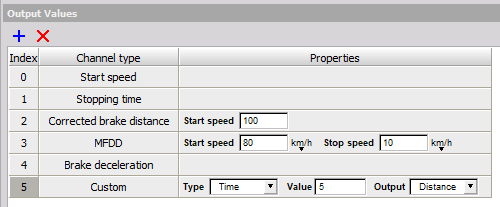# Brake test

## Software configuration

After opening the software and configuration of input channels (Analog/digital inputs, CAN, GPS,…), go to Ch. Setup (step 1 on the picture below), click on button More… (step 2) and add Brake test mathematic module (step 3).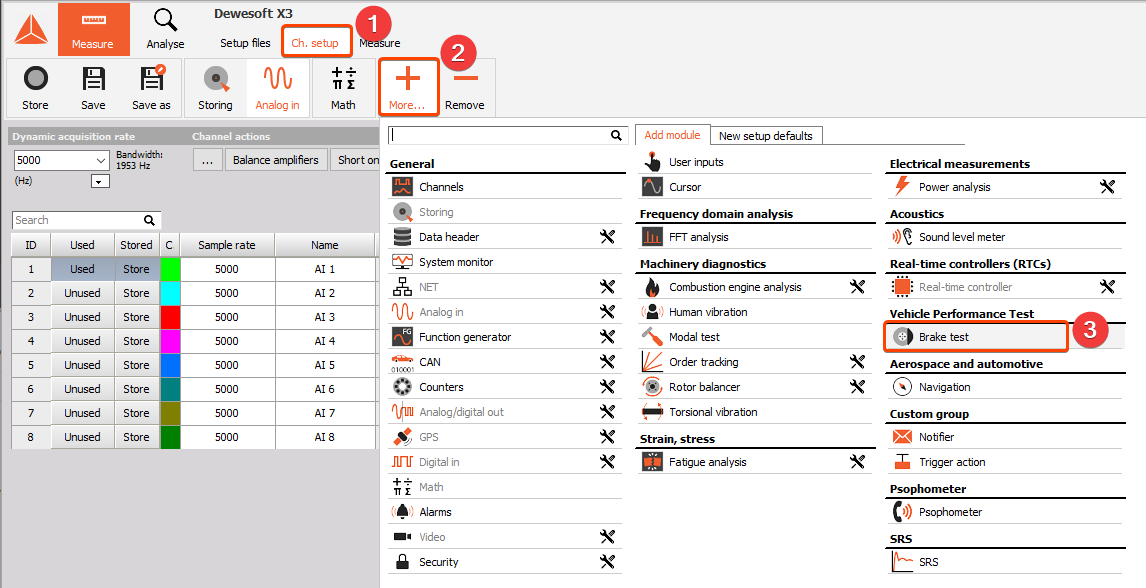## Brake test plugin

Here you can see the Brake test plugin setup form. All parameters for Brake test can be set here (Input channels, start and stop conditions, outputs,…).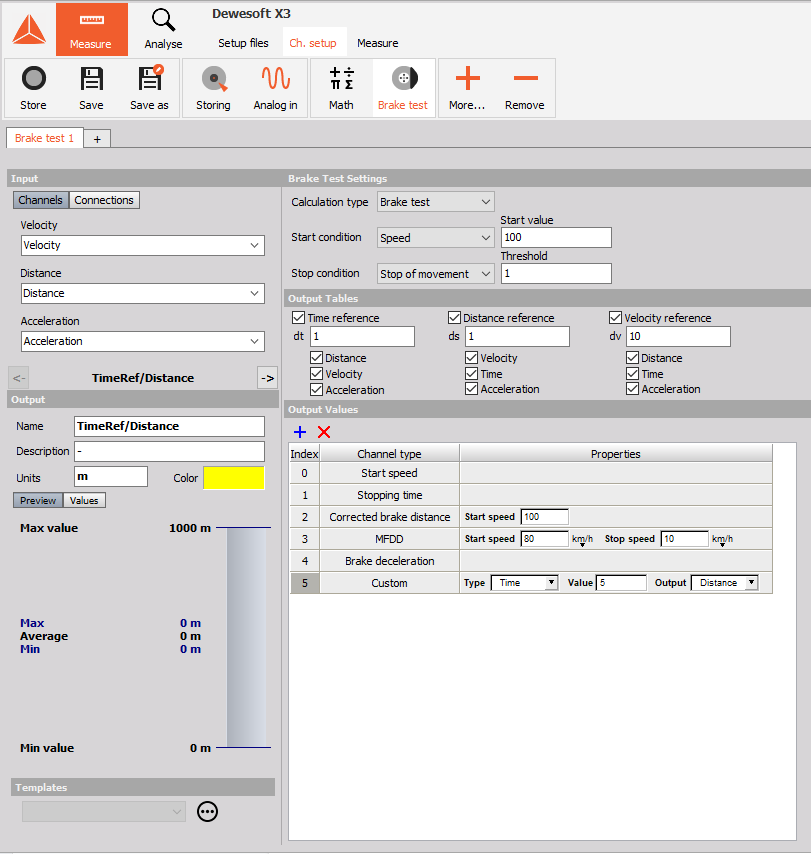### Input channels

Brake test plugin needs input data. Channels with input data are shown in the upper left part of setup form. Just search for appropriate channels in dropdown list and select it:

• Velocity (km/h),

• Distance (m),

• Acceleration (m/s2)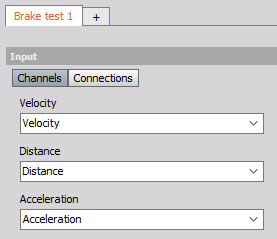Note: Correct units are very important inside Brake test plugin!

### Start and stop conditions

Start and stop conditions will define points where test is started and where it ends. But first the type of test (Brake or Acceleration) has to be selected.

Options for start conditions are:

• Speed – Start at selected speed

• Trigger – Start on trigger defined by channel and value

• Start of movement – Just for acceleration test, where additional threshold has to be defined

• Speed from channel – Start when speed passes the value from certain single value channel (used to change speed limit with header/sequencer)

Options for stop conditions are:

• Speed – Stop at selected speed

• Trigger – Stop on trigger defined by channel and value

• Distance – Stop at certain distance

• Time – Stop after some time

• Stop of movement – Stop when vehicle stops. Additional threshold parameter has to be defined to interpolate the data

• Speed from channel - Start when speed passes the value from certain single value channel (used to change speed limit with header/sequencer)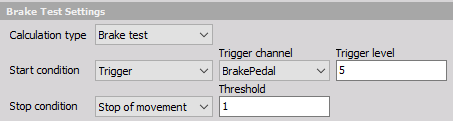### Measurement results

Next thing are the outputs. We have to define what we want to have as a result of the measurement. First there are tables which give more detailed information of test. They are called reference tables and they can give value of Velocity, Distance, Acceleration and/or Time at certain measure points, which are defined with Velocity, Distance or Time intervals. For example:

1. Time and distance on every 10km/h speed change (red square on picture below),

2. Speed for every 1 m travel (blue square),…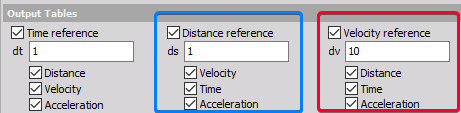In total there are 9 options and just check the ones you need.

Important note: Value has to be bigger than 0!

Then there are single values which are calculated from test data. Those are:

• Start speed – Speed at start of the test

• Stopping time – Total stopping time

• Corrected brake distance – Calculated brake distance corrected for distance start speed. It is calculated from actual brake distance multiplied by the ratio of the square of the actual start speed to the nominated correct distance start speed.

$\hspace{140pt}$ $\huge {CBD = \frac{(s_e - s_b) v^2_{CBD}}{v^2_b}}$

$s_e$ and $s_b$ are distance at start and at the end. $v_{CBD}$ is corrected start speed, $v_b$ is start speed.

• MFDD (Mean Fully Developed Deceleration) – is usually taken as average deceleration between 80 % and 10 % of the test start speed.
• Brake deceleration – This is average brake deceleration, calculated from start speed, stop speed and time
• Custom – Will give exact value of Distance, Speed or Time at certain point of test. For example when speed is 20 km/h or Time is 2s,… (Note: Only one additional Custom channel is allowed)
• Max. deceleration – Maximum deceleration during test, excluding last 0.5 sec.